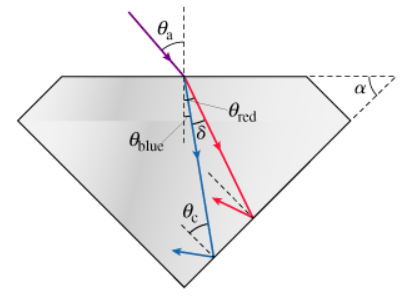# Problem: A beam of white light is incident on the surface of a diamond at an angle θa. (Figure 1) Since the index of refraction depends on the light's wavelength, the different colors that comprise white light will spread out as they pass through the diamond. The indices of refraction in diamond are nred = 2.410 for red light and nblue = 2.450 for blue light. The surrounding air has nair 1.000. Note that the angles in the figure are not to scale.Calculate vred, the speed of red light in the diamond. To four significant figures, c = 2.99x108 m/s. Express your answer in meters per second to four significant digits.

###### FREE Expert Solution

Speed of light in a medium:

$\overline{){\mathbf{v}}{\mathbf{=}}\frac{\mathbf{c}}{\mathbf{\eta }}}$###### Problem Details

A beam of white light is incident on the surface of a diamond at an angle θa. (Figure 1) Since the index of refraction depends on the light's wavelength, the different colors that comprise white light will spread out as they pass through the diamond. The indices of refraction in diamond are nred = 2.410 for red light and nblue = 2.450 for blue light. The surrounding air has nair 1.000. Note that the angles in the figure are not to scale.Calculate vred, the speed of red light in the diamond. To four significant figures, c = 2.99x108 m/s. Express your answer in meters per second to four significant digits.